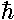## Square Wells p.13

### Three Dimensional Square Wells

We consider here a 3d rectangular "infinite square well". The potential is 0 inside a box with diagonal points of the origin and (Lx,Ly,Lz) and infinite outside the box. (Below we can only show 2-d.)(We use here the "alternative origin" rather having the box centered on the origin.) As before we have the "boundary" condition thatbe zero in the infinitely disallowed region outside the box. Inside the box, where the potential is zero, we have the Schrödinger's equation:Since the Hamiltonian is the sum of three terms with totally separate variables, we try a product wavefunction:=X(x)·Y(y)·Z(z)

As usual with separation of variables, the Schrödinger's equation separates into three terms, one only in x, the next only in y, the last only in z:These familiar differential equations have the usual solution:Now we apply our boundary conditionsThe resulting normalized wavefunctions are:It is difficult to plot of the probability density in 3-d. Consider a typical wavefunction: nx=2, ny=1, nz=3, in a box where (Lx,Ly,Lz) = (3,1,2). The probability density would be "diced" by nodal planes (i.e., planes on which the probability density is zero). In between the nodal planes, there would be local maxima for probability density. There would be one nodal plane parallel to the yz plane dividing the box along the x axis into two regions (nx=2). There would be no nodal planes parallel to the xz plane dividing the box along the y axis as ny=1. There would be two nodal plane parallel to the yz plane dividing the box along the z axis into three regions (nz=3). Click here for a virtual reality tour of such nodal planes in such a transparent box.

It is often convenient to describe wavefunctions in terms of "k-space" or "n-space", that is we label wavefunctions by k=(kx,ky,kx) vector or by n=(nx,ny,nz). In either case there is a lattice of points in the plane each one of which represents a possible wavefunctions. In the case of n-space, the lattice consists of those points with whole number x,y,z values. In the case of k-space, the lattice points are spaced by/Lx,/Ly,/Lz. (k-space goes by many different names. The momentum p=k is closely related to k, hence "momentum space". k is also closed related to "reciprocal lattice space". Since k=2/, k is often called a "wave number" [danger: the same thing without the 2is also called wave number].) The main idea for us is that E|k|2

Here is an energy level diagram showing the relationship between n=(nx,ny,nz) and the energy for a cube (Lx=Ly=Lz) square well.Click here for a (fairly gratuitous) virtual reality tour of the n-space lattice.

The red state (1,1,1) has the lowest energy followed by three sets of triplely degenerate states: orange (2,1,1), (1,2,1), (1,1,2); green (1,2,2), (2,1,2), (2,2,1); blue-green (3,1,1), (1,3,1), (1,1,3); a singlet: blue (2,2,2) and six degenerate violet states of the general form: (1,2,3). Note that higher energy states are further away from the origin.